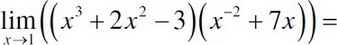# AP Calculus AB Practice Test 18

### Test Information10 questions20 minutes

Calculator Disallowed

1. Find the equation for the normal line to y = 3x2 - 6x at (2, 0).

2.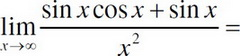3.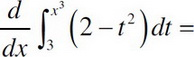4.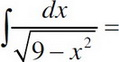5. Find for f′(x) = x3 + 2x when x = 1.

6.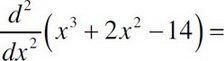7. Find the absolute maximum on the interval [-2, 2] for the curve y = 4x5 - 10x2 - 8.

8. Find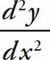at (-1, -2) if 3x3 - 2x2 + x = y3 + 2y2 + 3y .

9. Find the value of c that satisfies Rolle's theorem for f(x) = 2x4 - 16x on the interval [0, 2].

10.